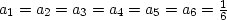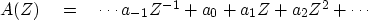Next: THE CENTRAL-LIMIT THEOREM Up: Resolution and random signals Previous: Speed of 2-D Gaussian

# PROBABILITY AND CONVOLUTION

One way to obtain random integers from a known probability function is to write integers on slips of paper and place them in a hat. Draw one slip at a time. After each drawing, replace the slip in the hat. The probability of drawing the integer i is given by the ratio ai of the number of slips containing the integer i divided by the total number of slips. Obviously the sum over i of ai must be unity. Another way to get random integers is to throw one of a pair of dice. Then all ai equal zero except.The probability that the integer i will occur on the first drawing and the integer j will occur on the second drawing is ai aj. If we draw two slips or throw a pair of dice, then the probability that the sum of i and j equals k is the sum of all the possible ways this can happen:(57)
Since this equation is a convolution, we may look into the meaning of the Z-transform(58)
In terms of Z-transforms, the probability that i plus j equals k is simply the coefficient of Zk in(59)

 The probability density of a sum of random numbers is the convolution of their probability density functions.

## EXERCISES:

1. A random-number generator provides random integers 2, 3, and 6 with probabilities p(2)=1/2, p(3)=1/3, and p(6)=1/6. What is the probability that any given integer n is the sum of three of these random numbers? (HINT: Leave the result in the form of coefficients of a complicated polynomial.)Next: THE CENTRAL-LIMIT THEOREM Up: Resolution and random signals Previous: Speed of 2-D Gaussian
Stanford Exploration Project
10/21/1998